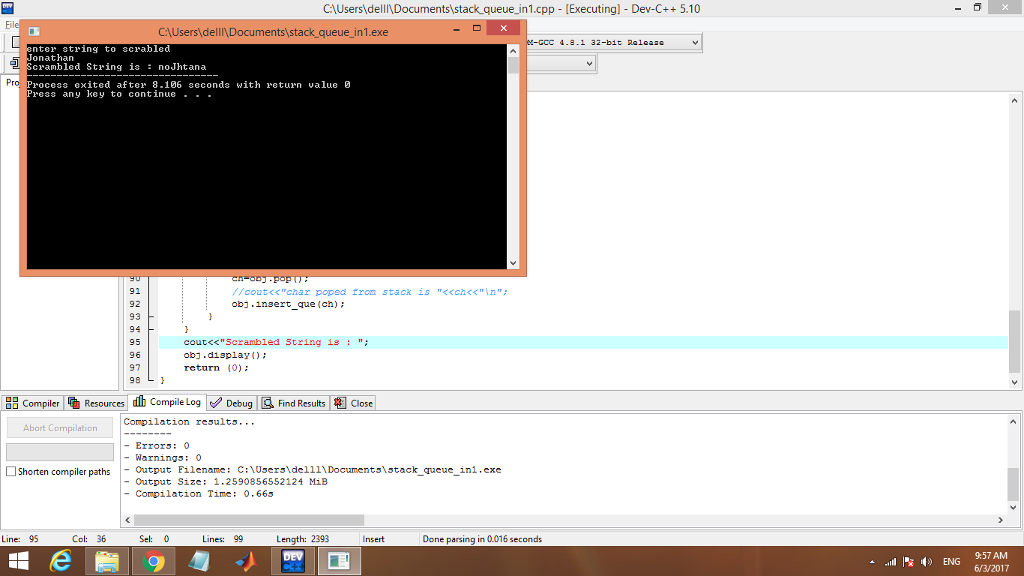# Answered! You will use a stack and a queue to implement a scrambled version of the rotational hashing function. Before…

You will use a stack and a queue to implement a scrambled version of the rotational hashing function. Before applying the rotational hash to the string, you will implement a function that will scramble the characters in the string so that every 3 successive characters in the string are inverted. For example, if the string to be hashed is “Jonathan”, then the function should turn the string into “noJhtana” before applying the rotational hash. This function can be easily implemented using a stack and a queue.

I have solved this problem by coding in C++. Both stack and queue are implemented using array data structure inside one class.

Logic:

Don't use plagiarized sources. Get Your Custom Essay on
Answered! You will use a stack and a queue to implement a scrambled version of the rotational hashing function. Before…
GET AN ESSAY WRITTEN FOR YOU FROM AS LOW AS \$13/PAGE

1.3 character are firstly pushed onto the stack by using the loop.

2. Then they are popped and inserted in the queue.

3. Finally, the result is shown from a queue.

—————————————————————————————————————————————————–

a.cpp

// stack queue in single program using array(character)
#include<iostream>
#include<string.h>
using namespace std;

// we have make a one class that implement operation of stack and queue
class stack_queue
{
char stk;
char queue;
int top,front,rear;
public:
stack_queue()
{
top=-1;
front=-1;
rear=-1;

}
// push in stack
void push(char x)
{
if(top==9)
{
cout <<“stack over flow”;
return;
}
stk[++top]=x;
// cout <<“inserted :” <<x<<“n”;
}
// pop from stack
char pop()
{
if(top==-1)
{
cout <<“stack under flow”;
}
return stk[top–];
}
// insert in queue
void insert_que(char x)
{
if(rear==29)
{
cout <<“Queue over flow”;
return;
}
else if(front==-1)
{
front=0;
rear=0;
}
else
{
rear++;
}
queue[rear]=x;
//cout <<rear<<” Queue inseted is ” <<queue[rear]<<“n”;
}

void display()
{
for(int i=0;i<=rear;i++)
cout <<queue[i];
}
};

int main()
{
stack_queue obj;
char str,ch;
int i,j,k,count,len;
cout<<“enter string to scrabledn”;
cin>>str;
len=strlen(str);

for(i=0;i<=len;i+=3)
{
count=0;
// loop for insertion in stack
for(j=i;j<(3+i)&&str[j]!=’’;j++)
{
obj.push(str[j]);
count++;
}
//loop to pop from stack and insert in queue
for(k=count;k>0;k–)
{
ch=obj.pop();
//cout<<“char poped from stack is “<<ch<<“n”;
obj.insert_que(ch);
}
}
cout<<“Scrambled String is : “;
obj.display();
return (0);
}

—————————————————————————————————————————————————–

Output: i have tested the same string as given in the question.